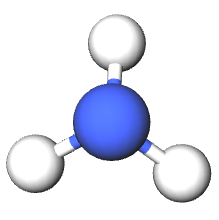# 14.4: The pH of Solutions of Weak Bases

The pH of a solution of a weak base can be calculated in a way which is very similar to that used for a weak acid. Instead of an acid constant Ka, a base constant Kb must be used. If a weak base B accepts protons from water according to the equation

$\text{B} + \text{ H}_{\text{2}}\text{O}\rightleftharpoons\text{BH}^{+} + \text{OH}^{-} \label{1}$

then the base constant is defined by the expression

$K_{b}=\dfrac{\text{ }\!\![\!\!\text{ BH}^{\text{+}}\text{ }\!\!]\!\!\text{ }\!\![\!\!\text{ OH}^{-}\text{ }\!\!]\!\!\text{ }}{\text{ }\!\![\!\!\text{ B }\!\!]\!\!\text{ }} \label{2}$

A list of Kb values for selected bases arranged in order of strength is given in the table below. This table is part of our larger collection of acid-base resources.

Table $$\PageIndex{1}$$: The Base Constants for Some Bases at 25°C.
Base Formula and Ionization Equation Kb Molecular Shape
Ammonia $$NH_3 + H_2O \rightleftharpoons NH^+_4 + OH^–$$ 1.77 × 10–5Aniline $$C_6H_5NH_2 + H_2O \rightleftharpoons C_6H_5NH^+_3 + OH^–$$ 3.9 × 10–10Carbonate ion $$CO_3^{2–} + H_2O \rightleftharpoons HCO^-_3 + OH^–$$ 2.1 × 10–4Hydrazine $$N_2H_4 + H_2O \rightleftharpoons N_2H^+_5 + OH^–$$
$$N_2H^+_5 + H_2O \rightleftharpoons N_2H_6^{2+} + OH^–$$
K1 = 1.2 × 10–6
K2 = 1.3 × 10–15Hydride ion $$H^– + H_2O \rightarrow H_2 + OH^–$$ largePhosphate ion $$PO_4^{3–} + H_2O \rightleftharpoons HPO^{2-}_4 + OH^–$$ 5.9 × 10–3Pyridine $$C_5H_5N + H_2O \rightleftharpoons C_5H_5NH^+ + OH^–$$ 1.6 × 10–9• Taken from Hogfelt, E. Perrin, D. D. Stability Constants of Metal Ion Complexes, 1st ed. Oxford; New Pergamon, 1979-1982. International Union of Pure and Applied Chemistry, Commission on Equilibrium. ISBN: 0080209580

To find the pH we follow the same general procedure as in the case of a weak acid. If the stoichiometric concentration of the base is indicated by cb, the result is entirely analogous to equation 4 in the section on the pH of weak acids; namely,

$K_{b}=\dfrac{\text{ }\!\![\!\!\text{ OH}^{-}\text{ }\!\!]\!\!\text{ }^{\text{2}}}{c_{b}-\text{ }\!\![\!\!\text{ OH}^{-}\text{ }\!\!]\!\!\text{ }} \label{3}$

Under most circumstances we can make the approximation

$c_b – [OH^–] \approx c_b$

in which case Equation $$\ref{3}$$ reduces to the approximation

$[OH^–] ≈ \sqrt{K_{b}c_{b}} \label{4}$

which is identical to the expression obtained in the acid case (approximation shown in equation 6 in the section on the pH of weak acids) except that OH replaces H3O+ and b replaces a. Once we have found the hydroxide-ion concentration from this approximation, we can then easily find the pOH, and from it the pH.

Example $$\PageIndex{1}$$: pH using Kb

Using the value for Kb listed in the table, find the pH of 0.100 M NH3.

Solution

It is not a bad idea to guess an approximate pH before embarking on the calculation. Since we have a dilute solution of a weak base, we expect the solution to be only mildly basic. A pH of 13 or 14 would be too basic, while a pH of 8 or 9 is too close to neutral. A pH of 10 or 11 seems reasonable. Using Eq. (4) we have

\begin{align}\text{ }\!\![\!\!\text{ OH}^{-}\text{ }\!\!]\!\!\text{ }=\sqrt{K_{b}c_{b}} \\ \text{ }=\sqrt{\text{1}\text{.8 }\times \text{ 10}^{-\text{5}}\text{ mol L}^{-\text{1}}\text{ }\times \text{ 0}\text{.100 mol L}^{-\text{1}}} \\\text{ }=\sqrt{\text{1}\text{.8 }\times \text{ 10}^{-\text{6}}\text{ mol}^{\text{2}}\text{ L}^{-2}}=\text{1}\text{.34 }\times \text{ 10}^{-\text{3}}\text{ mol L}^{-\text{1}} \\\end{align}

Checking the accuracy of the approximation, we find

$$\dfrac{\text{ }\!\![\!\!\text{ OH}^{-}\text{ }\!\!]\!\!\text{ }}{c_{\text{b}}}=\dfrac{\text{1}\text{.34 }\times \text{ 10}^{-\text{3}}}{\text{0}\text{.1}}\approx \text{1 percent}$$

The approximation is valid, and we thus proceed to find the pOH.

$$\text{pOH}=-\text{log}\dfrac{\text{ }\!\![\!\!\text{ OH}^{-}\text{ }\!\!]\!\!\text{ }}{\text{mol L}^{-\text{1}}}=-\text{log(1}\text{.34 }\times \text{ 10}^{-\text{3}}\text{)}=\text{2}\text{.87}$$

From which

pH = 14.00 – pOH = 14.00 – 2.87 = 11.13

This calculated value checks well with our initial guess.

Occasionally we will find that the approximation

cb – [OH] ≈ cb

is not valid, in which case we must use a series of successive approximations similar to that outlined above for acids. The appropriate formula can be derived from Eq. (3) and reads

[OH] ≈ $$\sqrt{K_{b}\text{(}c_{b}-\text{ }\!\![\!\!\text{ OH}^{-}\text{ }\!\!]\!\!\text{ )}}$$      (5)